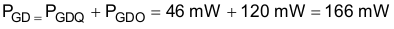SLUSCV6A April   2017  – February 2018

PRODUCTION DATA.

1. Features
2. Applications
3. Description
1.     Device Images
4. Revision History
5. Pin Configuration and Functions
6. Specifications
7. Parameter Measurement Information
8. Detailed Description
1. 8.1 Overview
2. 8.2 Functional Block Diagram
3. 8.3 Feature Description
4. 8.4 Device Functional Modes
9. Application and Implementation
1. 9.1 Application Information
2. 9.2 Typical Application
1. 9.2.1 Design Requirements
2. 9.2.2 Detailed Design Procedure
3. 9.2.3 Application Curves
10. 10Power Supply Recommendations
11. 11Layout
12. 12Device and Documentation Support
13. 13Mechanical, Packaging, and Orderable Information

• NPL|13

#### 9.2.2.4 Estimate Gate Driver Power Loss

The total loss, PG, in the gate driver subsystem includes the power losses of the UCC21225A (PGD) and the power losses in the peripheral circuitry, such as the external gate drive resistor. Bootstrap diode loss is not included in PG and is not discussed in this section.

PGD is the key power loss which determines the thermal safety-related limits of the UCC21225A, and it can be estimated by calculating losses from several components.

The first component is the static power loss, PGDQ, which includes quiescent power loss on the driver as well as driver self-power consumption when operating with a certain switching frequency. PGDQ is measured on the bench with no load connected to OUTA and OUTB at a given VCCI, VDDA/VDDB, switching frequency and ambient temperature. Figure 4 shows the per output channel current consumption vs. operating frequency with no load. In this example, VVCCI = 5 V and VVDD = 12 V. The current on each power supply, with INA/INB switching from 0 V to 3.3 V at 200 kHz, is measured to be IVCCI = 2 mA, and IVDDA = IVDDB = 1.5 mA. Therefore, the PGDQ can be calculated with

Equation 11.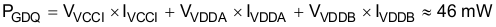The second component is switching operation loss, PGDO, with a given load capacitance which the driver charges and discharges the load during each switching cycle. Total dynamic loss due to load switching, PGSW, can be estimated with

Equation 12.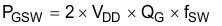where

• QG is the gate charge of the power transistor at VVDD.

If a split rail is used for turn on and turn off, then VVDD is the total difference between the positive rail to the negative rail.

So, for this example application:

Equation 13.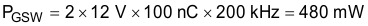QG represents the total gate charge of the power transistor switching 400 V at 14 A, and is subject to change with different testing conditions. The UCC21225A gate driver loss on the output stage, PGDO, is part of PGSW. PGDO will be equal to PGSW if the external gate driver resistances and power transistor internal resistances are 0-Ω, and all the gate driver loss will be dissipated inside the UCC21225A. If there are external turn-on and turn-off resistances, the total loss will be distributed between the gate driver pull-up/down resistances, external gate resistances, and power transistor internal resistances. Importantly, the pull-up/down resistance is a linear and fixed resistance if the source/sink current is not saturated to 4 A/6 A, however, it will be non-linear if the source/sink current is saturated. Therefore, PGDO is different in these two scenarios.

Case 1 - Linear Pull-Up/Down Resistor:

Equation 14.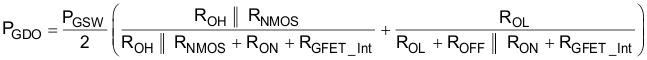In this design example, all the predicted source/sink currents are less than 4 A/6 A, therefore, the UCC21225A gate driver loss can be estimated with:

Equation 15.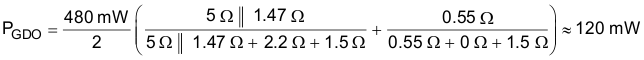Case 2 - Nonlinear Pull-Up/Down Resistor:

Equation 16.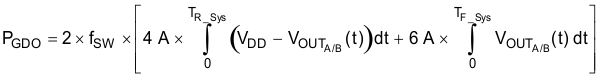where

• VOUTA/B(t) is the gate driver OUTA and OUTB pin voltage during the turn on and off period. In cases where the output is saturated for some time, this can be simplified as a constant current source (4 A at turn-on and 6 A at turn-off) charging/discharging a load capacitor. Then, the VOUTA/B(t) waveform will be linear and the TR_Sys and TF_Sys can be easily predicted.

For some scenarios, if only one of the pull-up or pull-down circuits is saturated and another one is not, the PGDO will be a combination of Case 1 and Case 2, and the equations can be easily identified for the pull-up and pull-down based on the above discussion.

The total gate driver loss dissipated in the gate driver UCC21225A, PGD, is:

Equation 17.